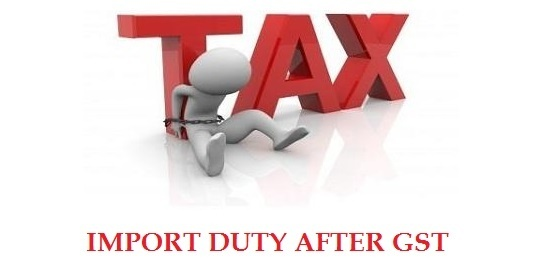With the advent of GST (Goods and Service Tax), the entire method of imposing import duty on products imported in India has been changed. Some upgraded duties and taxes after GST are basic custom duty, integrated goods & service tax and compensation cess. Here are two examples explained in order to easily understand the importance of GST and import duty after GST.Example 1- Hair Shampoo

How to calculate imposed import duty on Hair shampoo after GST?

Let’s suppose the assessable value (AS) of imported Hair shampoo in Into India is Rs300/-, basic custom duty (BCD) is 12.5% and IGST rate imposed (18%).

A) Basic customs duty on Hair Shampoo imported into India is 12.5% of 300 =Rs 37.5/-
B) IGST (Integrated Goods and Service Tax) is 18% of (AV + BCD) = 18% of 300+37.5 =Rs 60.75/-
C) Compensation Cess: 0% of (AV + BCD) = 0% of (300 + 37.5) = 0% of Rs 375.5/- = 0 (Nil)
D) Total tax imposed on imported Hair Shampoo= A+B+C =37.5 + 60.75 + 0 =Rs 98.95/-

Example 2- Pan Masala

How to calculate imposed import duty after GST on Pan Masala?

Let’s suppose the assessable value (AS) of imported Pan Masala in Into India is Rs200/-, basic custom duty (BCD) is 37.5%, IGST rate imposed (28%) and compensation cess is 60%.